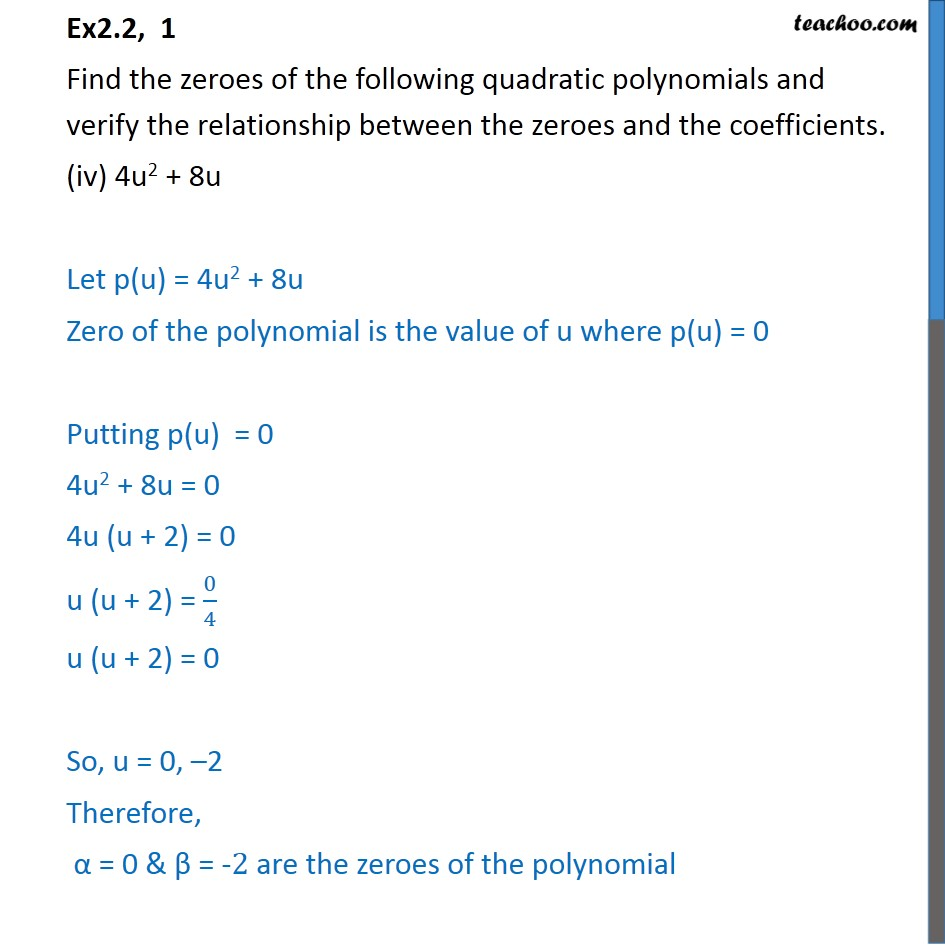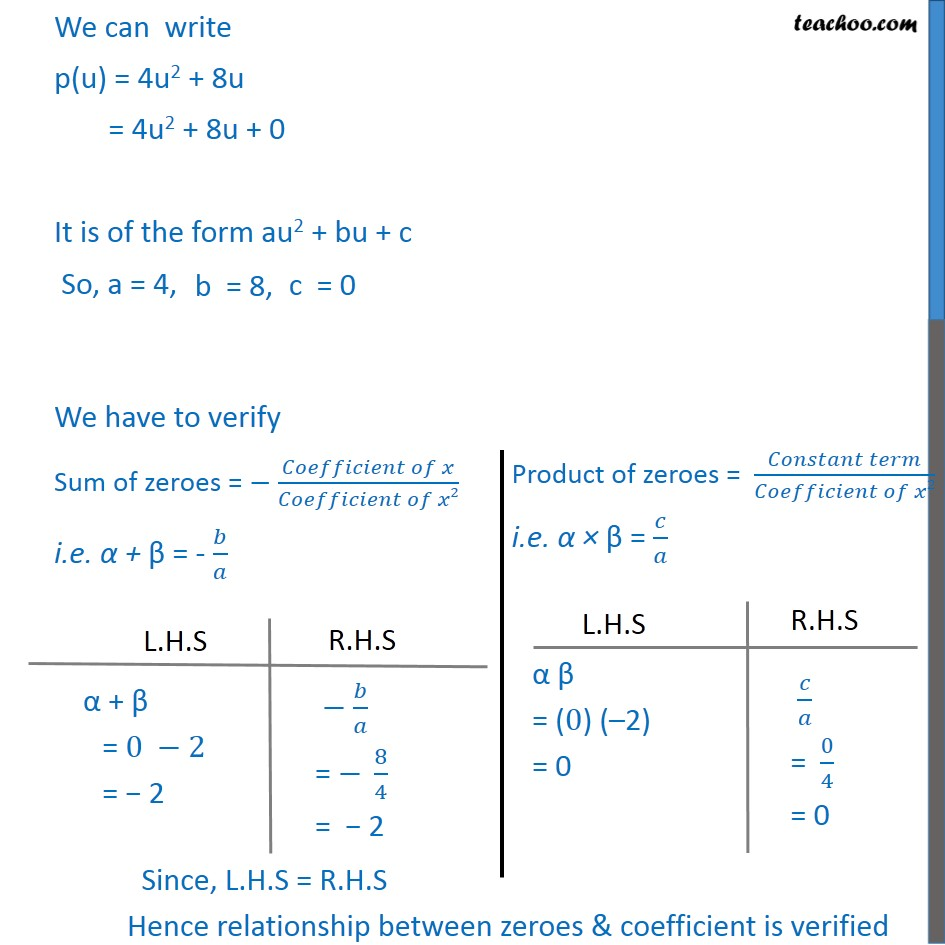1. Chapter 2 Class 10 Polynomials (Term 1)
2. Serial order wise
3. Ex 2.2

Transcript

Ex2.2, 1 Find the zeroes of the following quadratic polynomials and verify the relationship between the zeroes and the coefficients. (iv) 4u2 + 8u Let p(u) = 4u2 + 8u Zero of the polynomial is the value of u where p(u) = 0 Putting p(u) = 0 4u2 + 8u = 0 4u (u + 2) = 0 u (u + 2) = 0/4 u (u + 2) = 0 So, u = 0, –2 Therefore, α = 0 & β = -2 are the zeroes of the polynomial We can write p(u) = 4u2 + 8u = 4u2 + 8u + 0 It is of the form au2 + bu + c So, a = 4, We have to verify Sum of zeroes = − (𝐶𝑜𝑒𝑓𝑓𝑖𝑐𝑖𝑒𝑛𝑡 𝑜𝑓 𝑥)/(𝐶𝑜𝑒𝑓𝑓𝑖𝑐𝑖𝑒𝑛𝑡 𝑜𝑓 𝑥2) i.e. α + β = - 𝑏/𝑎